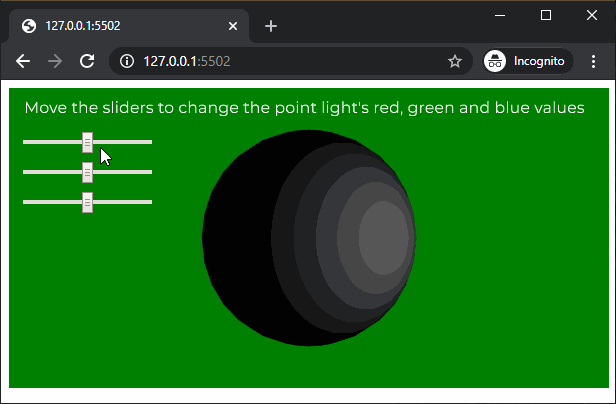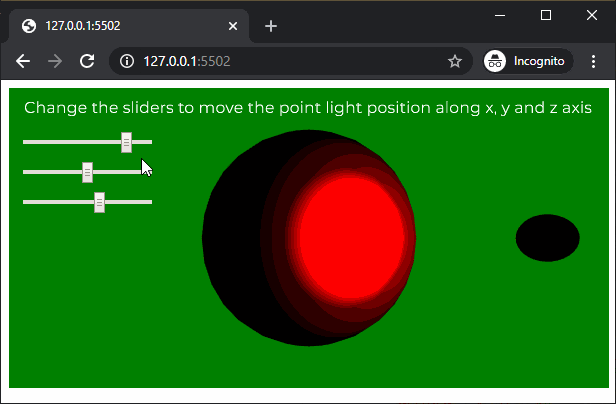# p5.js | pointLight() Function

• Last Updated : 27 Apr, 2020

The pointLight() function in p5.js is used to create a point lights with the specified color and position in the scene. A scene can have a maximum of 5 active point lights at a time.

Syntax:

`pointLight( v1, v2, v3, x, y, z )`

OR

`pointLight( v1, v2, v3, position )`

OR

`pointLight( color, x, y, z )`

OR

`pointLight( color, position )`

Parameters: This function accept eight parameters as mentioned above and described below:

• v1: It is a number which determines the red or hue value relative to the current color range.
• v2: It is a number which determines the green or saturation value relative to the current color range.
• v3: It is a number which determines the blue or brightness value relative to the current color range.
• x: It is a number which determines the x axis position of the light.
• y: It is a number which determines the y axis position of the light.
• z: It is a number which determines the z axis position of the light.
• position: It is p5.Vector which defines the position of the light.
• color: It is a color string or p5.Color which defines the color of the point light.

Below example illustrates the pointLight() function in p5.js:

Example 1:

 `let newFont;``let directionalLightEnable = ``false``;`` ` `function` `preload() {``  ``newFont = loadFont(``'fonts/Montserrat.otf'``);``}`` ` `function` `setup() {``  ``createCanvas(600, 300, WEBGL);``  ``textFont(newFont, 16);`` ` `  ``redSlider = createSlider(0, 255, 128, 1);``  ``redSlider.position(20, 50);`` ` `  ``greenSlider = createSlider(0, 255, 128, 1);``  ``greenSlider.position(20, 80);`` ` `  ``blueSlider = createSlider(0, 255, 128, 1);``  ``blueSlider.position(20, 110);``}`` ` `function` `draw() {``  ``background(``'green'``);``  ``text(``"Move the sliders to change the point light's red, "``+``                        ``"green and blue values"``, -285, -125);``  ``noStroke();`` ` `  ``redValue = redSlider.value();``  ``greenValue = greenSlider.value();``  ``blueValue = blueSlider.value();`` ` `  ``// Create a point light with the selected light color``  ``pointLight(redValue, greenValue, blueValue, 100, 0, 150);`` ` `  ``ambientMaterial(255);`` ` `  ``// Create the sphere on which the point light will work``  ``sphere(100);``}`

Output:Example 2:

 `let newFont;``let directionalLightEnable = ``false``;`` ` `function` `preload() {``  ``newFont = loadFont(``'fonts/Montserrat.otf'``);``}`` ` `function` `setup() {``  ``createCanvas(600, 300, WEBGL);``  ``textFont(newFont, 16);`` ` `  ``xPosSlider = createSlider(-150, 150, 100, 1);``  ``xPosSlider.position(20, 50);`` ` `  ``yPosSlider = createSlider(-300, 300, 0, 1);``  ``yPosSlider.position(20, 80);`` ` `  ``zPosSlider = createSlider(0, 250, 150, 1);``  ``zPosSlider.position(20, 110);`` ` `}`` ` `function` `draw() {``  ``background(``'green'``);``  ``text(``"Change the sliders to move the point light position "``+``                          ``"along x, y and z axis"``, -285, -125);``  ``noStroke();`` ` `  ``xPositionValue = xPosSlider.value();``  ``yPositionValue = yPosSlider.value();``  ``zPositionValue = zPosSlider.value();`` ` `  ``// Create a point light at the selected location``  ``pointLight(255, 0, 0, xPositionValue, yPositionValue, zPositionValue);`` ` `  ``// Create a sphere to show the demonstrate``  ``// of the point light location``  ``translate(xPositionValue, yPositionValue, zPositionValue);``  ``sphere(10);``  ``translate(-xPositionValue, -yPositionValue, -zPositionValue);`` ` `  ``specularMaterial(255);`` ` `  ``// Create the sphere on which the point light will work``  ``sphere(100);``}`

Output:Online editor: https://editor.p5js.org/

My Personal Notes arrow_drop_up